# 1st PUC Computer Science Model Question Paper 3 with Answer

Students can Download 1st PUC Computer Science Model Question Paper 3 with Answers, Karnataka 1st PUC Computer Science Model Question Papers with Answers helps you to revise the complete Karnataka State Board Syllabus and score more marks in your examinations.

## Karnataka 1st PUC Computer Science Model Question Paper 3 with Answers

Time: 3 Hours 15 Minutes
Max. Marks: 70

PART – A

I. Answer the following questions. (10 × 1 = 10)

Question 1.
Name the oldest computing tool.
Abacus is the oldest computing tool.

Question 2.
What are virtual keyboards?
A virtual keyboard is where a full-size image of a QWERTY keyboard is projected onto any surface. The letters are typed by touching the image of a key.

Question 3.
What is the important aspect of design of a solution?
The most important aspect of developing a solution is developing the logic to solve that specific problem.

Question 4.
Describe tokens.
The smallest individual units in a program are known as tokens.

Question 5.
What is an output stream?
Output stream is a sequence of characters from the program to an output device like a monitor or printer.

Question 6.
What is the other name for the ‘if-else’ statement?
The other name of the ‘if-else’ statement is two-way branching.Question 7.
Mention any one feature of subscript or index of an array.
Subscript or index of an array is always a positive whole number.

Question 8.
What is auser defined function?
A user-defined function is a self-contained block of statements, that performs a specific task, with a name given to it, defined by the user in a program.

Question 9.
What is the use of font dialog box?
Font dialog box contains the tool buttons like font name, font size, font bold, etc.,Question 10.
What is the use of an auto-fill option?
The auto-fill option can be used to insert a series of values in a range of cells easily.

PART-B

II. Answer any Five of the following questions. (5 × 2 = 10)

Question 11.
Expand IBM. What was the earlier name of IBM?
The expansion of IBM is “International Business Machine”. The earlier name of IBM was Tabulating Machine Company.

Question 12.
Expand the term MICR and BCR.
MICR:
BCR:

Question 13.
Define operating system. Give examples for operating systems.
An operating system or OS is a software program that enables the computer hardware to communicate and operate with the computer software. Examples for operating systems are Linux, MS-DOS, Windows 8, etc.

Question 14.
Define coding. What is a pseudo code?

• The process of representing an algorithmic solution or a flowchart solution Into a set of instructions in a programming language is called coding.
• Pseudocode is structured English, that consists of short, English phrases used to explain specific tasks within a program’s algorithm.

Question 15.
Which programming approach does the OOP follow? What is the meaning of abstraction in OOP?
OOP follows bottom-up approach of programming. Abstraction in OOP is “A model of a complex system that includes only the details essential to the perspective of the viewer of the system.”

Question 16.
Give the features of ‘char’ data type.

• C++ offers a predefined data type ‘char’ which is one byte in size and which can hold exactly one character such as an ‘a’ or ‘A’.
• To declare a variable of type char is char ch;Question 17.
Write any two library functions of stdlib.h.
Two library functions of stdlib.h are atoi() and itoa().

Question 18.
What is the difference between normal view mode and page layout view mode?
If the document consists of both graphical pictures and text then in the normal mode graphical picture cannot be displayed. They can be displayed only in the page layout mode.

PART – C

III. Answer any Five of the following questions. (5 × 3 = 15)

Question 19.
Give different types of computer monitors.
The different types of computer monitors are CRT monitors, LCD monitors, TFT monitors and LED monitors.

Question 20.
What is l’s complement? Give an example.
Representing a signed number with 1s complement is done by changing all the bits that are 1 to 0 and all the bits that are 0 to 1. For example, 1s complement of 1010100 is 0101011.

Question 21.
Write any three functions of an operating system.
1. Memory management:
The operating system allocates memory for requesting a process and frees the memory when the process is completed.

2. Device management:
The operating system is responsible to allocate input and output devices as and when they are required by the process.

3. Management of input/output:
The operating system allows control of access of programs to material resources via drivers.

Question 22.
What are sequential construct, selection, and iteration?
1. Sequential construct:
The ability’ of executing the program statements one after another in sequence is called sequential construct.

2. Selection:
The process of selecting certain set of statements based on a requirement for – execution is called selection.

3. Iteration :
It is the process of repeating the execution of certain set of statements repeatedly until a requirement is satisfied.Question 23.
What are floating-point constants, octal constants, and hexadecimal constants?
1. Floating-point constants:
They are numeric values that contain decimal points and can contain exponents.

2. Octal constants:
They are a sequence of digits starting with 0 (zero) to 7.

They are a of sequence of digits from 0 to 9 and A, B, C,D, E, F alphabet symbols that represent decimal numbers 10, 11, 12, 13, 14, and 15 respectively, preceded by ox or OX.

Question 24.
Explain manipulators.

1. A manipulator in a C++ is used to control the formatting of output and/or input values.

2. Manipulators can only be present in Input/Output statements. The end1 manipulator causes a newline character to be output.

3. end1 is defined in the <iostream> header file and can be used as long as the header file has been included.

Question 25.
Give explanation of declaration of one dimensional array with an example.
int marks;

• Name of the array is marks.
• Type of the array is integer.
• Size of the array is 6. i.e., we can store up to 6 integer values in the array.

Question 26.
Write the declaration syntax of a structure definition.
struct <structure-tag>
{
<data_type> <member variable 1>
<data_type> < member variable2> list of members
<data_type> < member variable3>
} <structure-variable-name1>, <structure-variable-name2>;

PART- D

IV. Answer any Seven of the following questions. (7 × 5 = 35)

Question 27.
Draw the schematic block diagram of a computer showing its essential components. Discuss the function of each component.a. Input Unit:

• It is a device through which we enter program and data into the computer. The data is then stored and processed with the help of programs.
• Central Processing UnitrThe Central Processing Unit (CPU)is divided into three units namely, the control unit, the arithmetic and logic unit and the memory unit.

b. Control Unit:
This unit is considered to be the nerve centre of the computer. It controls all arithmetic operations to be performed. It also coordinates the functions of all the hardware units of the computer.

1. Arithmetic and Logic Unit (ALU):
This unit performs all the arithmetic calculations and logical operations involved in a program.

2. Memory Unit:
The function of this unit is to store data and the set of instructions given by the programmer. The computation results are stored in the memory and they may be used for subsequent computations or sent to the output unit.

3. Output Unit:
The output unit receives information from the CPU and presents it to the user in the desired form. Some common output devices are visual display unit (VDU) and Printer.Question 28.
Write a short note on binary arithmetic.
Binary arithmetic is an essential part of all the digital computers and many other digital systems.
It is a key for binary subtraction, multiplication, division. The four rules of the binary addition are:
0 + 0 = 0
0 + 1 = 1
1 + 0 = 1
1 + 1 = 0 with a carry bit 1 112

Binary Subtraction:
Subtraction and borrow, these two words will be used very frequently for the binary subtraction. The four rules of the binary subtraction are:
0-0 = 0
0-1=0 with a borrow 1
1- 0=1
1-1 = 0

Question 29.
Explain the characteristics of algorithm.
The characteristics of algorithm are:

1. It must be simple.
2. Every step should perform a single task.
3. There should not be any confusion at any stage.
4. It must involve a finite number of instructions.
5. It should produce at least one output.
6. It must give a unique solution to the problem.
7. The algorithm must terminate and must not enter to infinity.

Question 30.
Write a note on character set and tokens. Give example.
Character set is a set of valid characters that a language can recognize.

• Letters A-Z,
• Digits 0-9
• Special characters + – * / A \ ()[]{} = != o ‘ @

Tokens:
A token is a group of characters that logically belong together. C++ uses the following types of tokens. Keywords, Identifiers, Literals, Punctuators, and Operators.

Question 31.
Explain the structure of a C++ program with an example.The various parts of the above program:

• headers, which contain information that is either necessary or useful to program. For this program, the header <iostream> is needed.
• The next line // main() is where the program execution begins. It is a single-line comment available in C++. Single-line comments begin with // and stop at the end of the line.
• The line int main() is the main function where the program execution begins.
• The pair of { } indicates the body of the main function.
• The next line cout>> “This is my first C++ program.”; causes the message “This is my first C++ program” to be displayed on the screen.
The next line return 0; terminates main( )function and causes it to return the value 0 to ‘ the calling process.Question 32.
Explain switch-case statement with a suitable program example.
Switch statement compares the value of an expression against a list of integers or character constants. The list of constants is listed using the “case” statement along with a “break” statement to end the execution.Result:
Enter the day of the week between 1-7::7
Sunday
In the above Control Structure example, the “switch” statement is used to find the day of the week from the integer input got from the user. The value present in the day is compared for equality with constants written in the word case. Since no equality is achieved in the above example (from 1 to 6) as the entered value is 7, default value is selected and gives “Sunday” as the result.

Question 33.
Write a program to find the sum of all the digits of a number using while statement.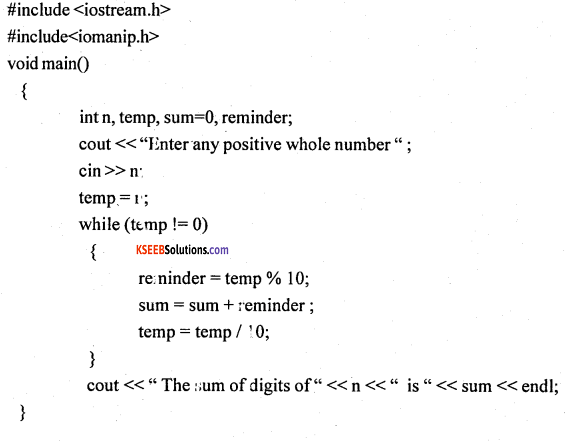Question 34.
Write a C++ program to find the second largest out of the n numbers in the array.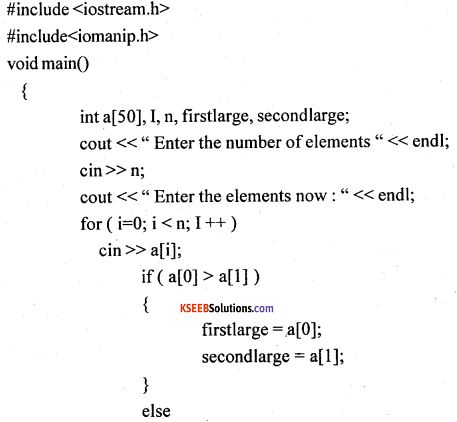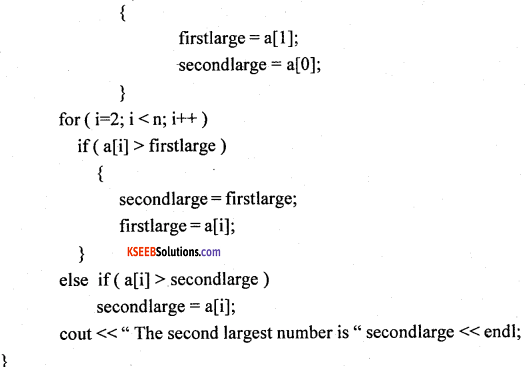Question 35.
Explain passing default argument to the function with an example.
When we define a function, we can specify a default value for each of the parameters. This value will be used if the corresponding argument is left blank during function call to the called function.

This is done by using the assignment operator and assigning values for the arguments in the function definition. During the function call, if a value for that parameter is not passed then default value is used, but if a value is mentioned then this default value is ignored and the passed value is used.

Consider the following example: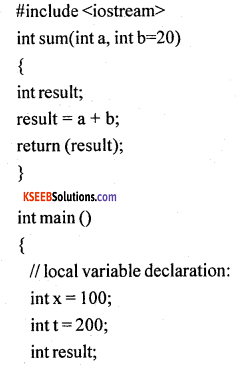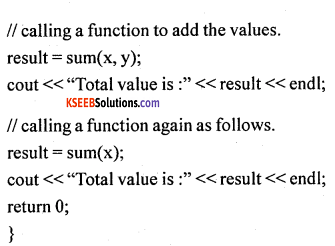In the above program, the statement int sum(int a, int b=20) in the function header indicates the v default argument to the variable b is assigned with the value 20. During the first function call, the statement result = sum(x, y); copies the value of x and y to the called function and process of the result.

During second time function call i.e., result = sum(x);, only one argument is mentioned and the value for second argument is obtained from the default argument which is mentioned in the function definition i.e., b=20 and gives the processed result.

Question 36.
Mention “the #Steps to add rows, columns, to change row size, column width and delete a cell in a worksheet.

1. The steps to add rows: select the row → insert → rows
2. The steps to add columns: select the column → insert →• columns
3. The steps to change row size: select cell → format → row height → height in numbers
4. The steps to change column width: select cell → format → column width → width in numbers
5. The steps to delete a cell: Move the cell pointer to the cell to be deleted → mouse right-click → delete → shift cell left/ upQuestion 37.
Write a short note on internet.
Internet is a worldwide network of computers connecting thousands and thousands of com¬puters across the globe. It is formed by the joining of many smaller networks around the world to form the largest network in the world.

In 1969 the American Department of Defense (DOD) started a network of devices called ARPANET (Advanced Research Projects Administration Network) with one computer in California and three in Utah. The actual term Internet was coined in 1995 by the FNC (Federal Networking Council, USA).

The computers of the Internet are connected through telephone lines, satellite links, modem and through many other means.

Internet consists of the following applications:

• World Wide Web # E-Mail # Chatting # Video Conferencing
• Searching for information # Online Shopping and Trade # Education and Research Uses

of internet:

• One can talk to anyone anywhere around the world.
• Ocean of resources waiting to be mined.
• One can do research on any subject for a project.
• Online business, on-line reservations can be done sitting at home or workplace.
error: Content is protected !!